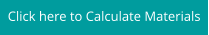# Gravel

Guadalupe Gravel ⅜”, #57, 1 ½”, 1 ¾”, 1”-3”, 1-3”, ⅜” Premix and 1” Premix### Calculations

Square or Rectangle: Length (in feet) x Width (in feet) x Depth (in inches) /324 = cubic yards Circle: 3.14 x Radius (in feet) x Radius (in feet) x Depth (in inches) /324 = cubic yards Triangle: Base (in feet) x Height (in feet) x Depth (in inches) /648 = cubic yards Oval: Length (in feet) x Width (both at midpoint in feet) x 0.8 x Depth (in inches) /324 = cubic yards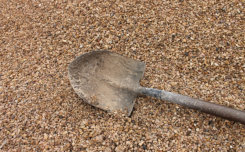### Gravels, Sands and Soil⅝”, 1 ½”, 3-5”

⅝”, 1 ½”, 3-5”

### Nueces Gravel 1”

5/8”,1”, 2”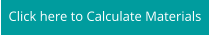### to dust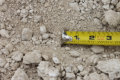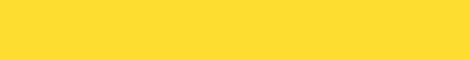# Gravel

Guadalupe Gravel ⅜”, #57, 1 ½”, 1 ¾”, 1”-3”, 1-3”, ⅜” Premix and 1” Premix### Calculations

Square or Rectangle: Length (in feet) x Width (in feet) x Depth (in inches) /324 = cubic yards Circle: 3.14 x Radius (in feet) x Radius (in feet) x Depth (in inches) /324 = cubic yards Triangle: Base (in feet) x Height (in feet) x Depth (in inches) /648 = cubic yards Oval: Length (in feet) x Width (both at midpoint in feet) x 0.8 x Depth (in inches) /324 = cubic yards### Soil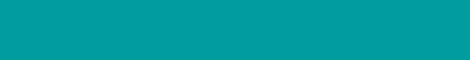⅝”, 1 ½”, 3-5”

⅝”, 1 ½”, 3-5”

### Nueces Gravel 1”